Maths-
General
Easy

Question

# from the graph below, identify . P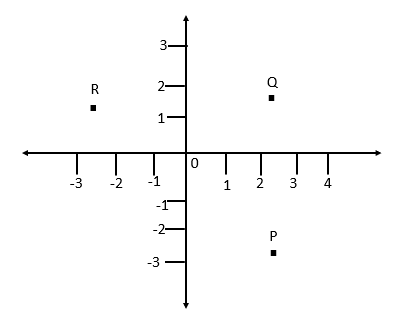## (-2,-1)(2,-2)(0,0)(4,-2)Hint:

## The correct answer is: (2,-2)

### Step1: Locating point of the graph.To get to point we will first take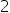steps on the positive x-axis as point P is just lying the above the pointof x-axis. After that we will takein the negative y-axis as the point P is lying along the negative y-axis.Step2: Finding the coordinatesX-Coordinate of point P will be =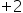, because we are going on the positive x-axisY-Coordinate of point P will be =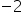, because we are going on the negative y-axis.So, the Coordinates of the point P will be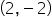#### With Turito Foundation.#### Get an Expert Advice From Turito.